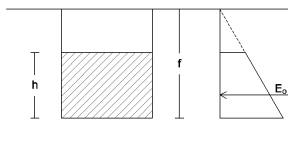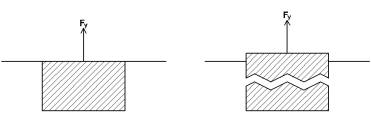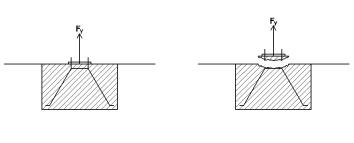##### IntroductionThis is the simplest case. The foundation is subject to the vertical pulling load  Fy. To provide stability, the foundation will resist this force in several ways:

• Rw by its own weight
• Rp by the weight of the pavement that may exist on the foundation
• Rf by the lateral friction against the ground
• Rs by the shear strength by the slab of pavement, if any.

Let’s study each of these stabilizing factors.

##### Self-weight

The simplest standard foundation consists of a prism of concrete, lightly reinforced, with dimensions a, b and h, located at an a depth f, where:

• a , b  horizontal dimensions of the footing
• h  height of the footing
• f  distance between the base of the foundation and the level of the ground.

If the footing is levelled to the soil surface, then  f = h

Concrete density of the foundation γc is a value between 2.2 and 2.5, depending on the amount of reinforcement. If we take a conservative value we can use a density of 2.3.

If the foundation does not reach the surface, and the hole is overfilled with soil of density γt, it will be necessary to add the weight of this soil.

Thus, the resistance due to the foundation self-weight will be

Rw = a · b · (h · γc + (f – h) · γt)

##### Friction

Friction is the force generated at the lateral sides of the footing at the moment that pulling force surpasses the footing self weight and it would begin to move up. Friction forces between two surfaces (concrete and soil) depend on two factors:

• Normal force (perpendicular) to the contact plane.
• Friction coefficient between two surfaces.

Normal force between these two surfaces is earth pressure at rest: soil against footing. If the footing is levelled to the soil surface, total value of earth pressure at rest, placed at 2h/3 distance from the footing surface, is:

E0 = ½ · K0 · γ . h²If the footing is deeper (f > h), this value increases to

E0 = ½ · K0 · γ . (f² – (f – h)²)

and the point where this force E0 is applied is the centre of gravity of the trapeze created by the forces against the footing

f – [1/3 · (f³ – (f – h)³) / f²]

So, if we have a prismatic footing a·b·h, with its top levelled to natural soil surface and this soil with certain characteristics  (γ,φ), extracting force due to friction at the four sides of the footing will be:

Rf = 2 · (a + b) · ½ · K0 · γ · h² · cf

where  cf  is the friction coefficient between soil and concrete. This coefficient can not be greater than the tangent of the angle of  internal friction of the soil.

It has already been said that in normal conditions (on site placed concrete) a value of  cf = tg(2φ/3) is accepted, although we must be very careful since this value can be zero in several occasions.

##### Pavement

When the foundation is covered by a pavement, placed on the ground, it will be necessary to evaluate the collaboration of this element.(The same comment could be made in case the footing is not levelled to the ground and has a thickness of soil above)

If it is a discontinuous element, unable to react as a “whole” to the extraction force, we can only consider as contribution the weight of the pavement above the foundation. In the case we have discussed so far, a footing which dimensions are a · b · h, the resistance Rp will be<

Rp = a · b · Qp

Being Qp the weight of the pavement per unit area (also counting the fixing elements: mortar, sand, etc.).

However, if the pavement contains a continuous slab (usually of reinforced concrete) the resistance to the extraction force Rs will be much higher, since not only the weight of the pavement contributes but it is necessary to add the shear strength of the slab, around the perimeter of the foundation.

Rs ≤ 2 · (a + b) · d · τ

##### Total resistance

As a conclusion and returning to the beginning of this section, the resistance of a particular foundation to a positive vertical force (extraction) depends on a series of values that can be summarized in this list:

• Dimensions of foundation, usually a, b, h (in the case of a pile: ø, h)
• Characteristics of the soil surrounding the foundation: earth pressure at rest coefficient K0, density γ, internal friction angle φ and cohesion C
• Roughness of soil-foundation contact
• If there is pavement above the foundation
• Total pavement weight (per unit area)
• If there is a reinforced concrete slab
• Thickness of the slab
• Shear strength τ of the concrete
• Bending strength of the slab
• Total weight of the slab. The final value being equal to

RT = Rw + Rf + Rp + Rs

##### Safety factor

As in any structural analysis, it is always necessary to maintain a safety margin between the applied actions and the resistance of our designs: the safety factor fs. In the present case it could not be otherwise. There are no regulations that define a specific safety factor for this problem, but common sense suggests that we use values about 1.5 or higher.

One possibility, always on the safety side, is to consider that the extraction force must be compensated exclusively by self weight (whether from the foundation, from the soil or from the pavement above the foundation), leaving all other resources (friction, shear force of the pavement slab, etc.) just to comply with the decided safety factor.

Rw + RP ≥ Fy
RT ≥ fs · Fy

We believe this is a very sensible criterion and we recommend following it in most cases. It is necessary to be very sure of the other contributions Rf and Rs to take them into account when compensating a vertical extraction force.

##### Constructive design

Taking into account that normally footings are designed for compressive loads (which push the foundation downwards), when referring to extraction loads it is necessary to take care about a very important fact: The reinforcement of the footing must be arranged in such a way to avoid horizontal rupture of the concrete footing, since in this case the weight of the foundation would be lower and could not compensate the pulling force Fy.A simple arrangement consists of carrying, in an inverted V, the reinforcement from the anchor point to the base of the foundation, as shown in the previous sketch.
It is not necessary to put a rebar grid at the bottom, as we usually do in many foundations, since the concrete of this zone is compressed, due to the tension of the reinforcement in form of inverted V.
Also, if the reinforcement of the foundation does not directly connect to the anchoring elements that resist the pulling force (cable, turnbuckles, etc.), it is necessary to check that the fastening elements (anchors, bolts, etc.) can not cause rupture of the concrete, separating the fixation from the rest of the foundation.##### Examples

Case 1

It is about designing a foundation capable of supporting a vertical pulling force Fy of 3 t.

Soil data, on which the foundation is located are:

Soil density γ = 1,9 t/m³
Internal friction angle of the soil φ = 35º
Roughness of the side faces of the footing = normal
Density of concrete = 2.3
cf = tg (2φ / 3) = 0.43
K0 = 0.4

We assume that the footing is located under a continuous pavement (limited surface but large enough) consisting of a reinforced concrete slab  d = 10 cm  thick, mortar and concrete tiles, with a total weight of  Qp = 450 kg/m².

We presume a  1 x 1 m(plan) footing. The weight of the pavement to be considered will be:

Rp = a · b · Qp = 1 · 1 · 0,45 = 0,45 t

If we want the total self-weight to balance the pulling force, it will be necessary that the foundation weight completes the amount of 3 t.

Rw = 1 · 1· h · 2,3 = 3 – 0,45 = 2,55 t
h = 1,1 m

We know that the concrete footing size will be  1 x 1 x 1.1 m. Now it is necessary to verify that the lateral friction and the shear of the concrete slab are sufficient to have a safety factor of  1,5. The friction resistance will be

Rf = 2 · (a+b) · ½ K0 · γ · h² · cf = 0,79 t

Therefore the contribution of the shear resistance Rs of the pavement slab will need to complete what is missing, up to the value of extraction modified by the safety factor: fs · Fy = 1,5 · 3 = 4,5 t

Rw + Rp + Rf = 3,79 t
Rs = 4,5 – 3,79 = 0,71 t
Rs = 2 · (a + b) · d · τ = 0,71 t
τ = 0,71 / 0,4 = 1,78 t/m² = 0,178 kg/cm²

which is a shear strength value perfectly suitable for any type of concrete.

Case 2

Let’s suppose the same example above, but in this case we assume that there is no pavement on the foundation. We can think of two possibilities:

2 a)
Friction between foundation and soil can be affected by rain or shrinkage.

It is a fairly typical case, especially in clayey soil. Whether it rains (the wet clay slips on the foundation wall) or drought and the ground is retracted (the floor separates and does not touch the foundation wall), we can not count on the resistance of the lateral friction of the foundation.In this case the weight of the foundation must be able to equal the value of extracting force (safety factor applied):

Rw = fs · Fy = 1,5 · 3 = 4,5 t

If we continue considering a footing which base is 1 x 1 will require a height  h  such that

h = 4,5 / 2,3 = 1,95 m

Probably it will be much more convenient, from the constructive point of view, to make an approximately cubic footing

a = b = h = √ (4,5 /2,3) = 1,25 m

2 b)
We can continue counting on the lateral friction of the footing, since soil is not shrinkable and it doesn’t lose friction when gets wet.

In this case, it is interesting to have a deep foundation, as this will increase the friction surface and the force (it depends on the square of h).

If we continue considering a footing plan of  1 x 1 m, we have already seen in case 1 that we did not have enough with a footing depth of  1.1 m, not even with the own weight of the pavement. We also know that without friction we would need a depth of  1,95 m. Therefore we will test it with an intermediate value:  h = 1,5 m.

Rw = 1 · 1· 1,5 · 2,3 = 3,45 t

It is then necessary that the friction completes the total resistance up to fs · Fy = 4,5 t

Rf ≥ 4,5 – 3,45 = 1,05 t
Rf = 2 · (a+b) · ½ K0 · γ · h² · cf = 2 · 0,4 · 1,9 · 1,5² · 0,43 = 1,47 t

In fact, the value obtained is higher than what was needed. Therefore a  1 x 1 x 1,5 m footing will be sufficient to withstand the extraction force of 3 t.

If  h = 1,4 then

Rw = 3,22 t ; Rf = 1,28 t >> Rw + Rf = 4,5

Which is exactly the needed value, and therefore, too adjusted for a normal analysis and mustn’t be accepted.